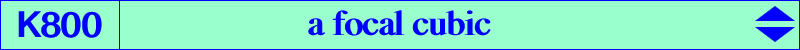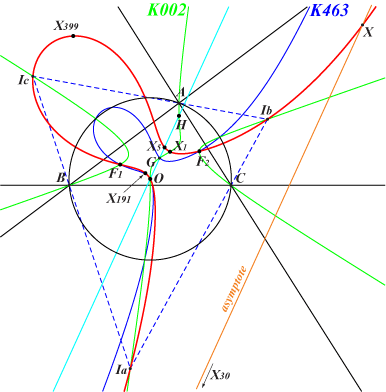too complicated to be written here. Click on the link to download a text file.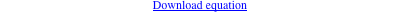X(1), X(3), X(5), X(30), X(191), X(399), X(5127), X(5535), X(6326), X(7701), X(18865) X(39162), X(39163), X(39164), X(39165) : foci of the Steiner inellipse excenters other points belowK800 is a focal cubic, locus of contacts of tangents drawn through its singular focus X(399) to the circles passing through X(3), X(5). Hence its orthic line is the Euler line. See also K1092, K1288, K1289. K800 is the cubic K464 for the excentral triangle since X(1263), X(5), X(143) are X(399), X(3), X(5) of the orthic triangle respectively and since the orthic triangle of the excentral triangle is ABC. K800 is also related to the pencil of focal cubics generated by K463 and the union of the line at infinity (twice) with the line X(3)X(110). See the generalization in K725, another member of the pencil and also K798, K799. K800 is the image of K798 under the homothety h(X3, 2). K800 is a member of several pencils of cubics, those generated by : • the Neuberg cubic K001 and the union of the line at infinity with the Stammler hyperbola passing through X(1), X(3), X(6), X(155), X(159), X(195), X(399), X(610), X(1498), X(1740), X(2574), X(2575), X(2916), X(2917), X(2918), X(2929), X(2930), X(2931), X(2935), X(2948), X(3216), etc. This pencil contains K913 and a central cubic with center O. Every cubic has its singular focus on the line X(3), X(74), X(110), X(399), etc. • K038, K187, K463 and the union of the line at infinity with the rectangular hyperbola with center X(2) passing through X(3), X(6), X(381), X(599), X(2574), X(2575). All these cubics pass through the four foci of the Steiner inellipse. • K725 and the union of the line at infinity with the rectangular hyperbola passing through X(3), X(4), X(195), X(576), X(1147), X(2574), X(2575), X(2888), X(2904). Note that all these hyperbolas pass through X(3) and are homothetic to the Jerabek hyperbola.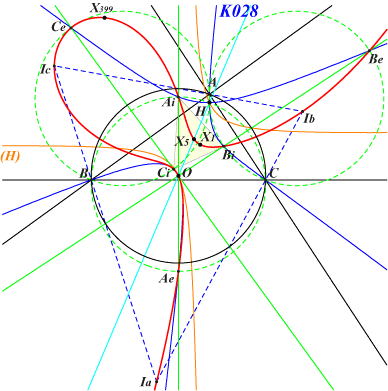K800 meets the perpendicular bisectors of ABC at X(3) and three pairs of points such as Ae, Ai lying on the circles passing through X(4) and the two end-points of the corresponding sides of ABC i.e. the reflections of the circumcircle of ABC about its sidelines. AiBiCi and AeBeCe are the 1st and 2nd Fuhrmann triangles respectively. K028 and K800 meet at these six points and X(3) which is a triple common point with tangent passing through X(399). The two cubics have the same polar conic at X(3) – hence the same osculating circle – which is the rectangular hyperbola (H) with center X(5) passing through X(3), X(4), X(195), X(576), X(1147), X(2574), X(2575), X(2888), X(2904) hence homothetic to the Jerabek hyperbola. (H) meets K800 at X(3) twice and four other points two by two symmetric about X(5) and lying on two perpendiculars at X(5) to the asymptotes of the rectangular circum-hyperbola passing through X(140), the midpoint of X(3)-X(5).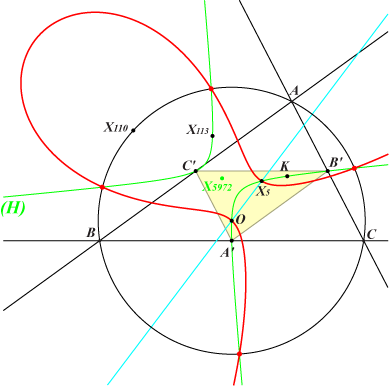K800 meets the circumcircle at four finite points which lie on the conic (H) which is the complement of the Jerabek hyperbola. (H) passes through X(3), X(5), X(6), X(113), X(141), X(206), X(942), X(960), X(1147), X(1209), X(1493), X(1511), X(2574), X(2575), X(2883), etc. Its center is X(5972) = X(125)-medial. (H) is : • the bicevian conic C(X2, X110), • the X(2)-Ceva conjugate of the Brocard axis, • the locus of centers of circum-conics whose axes are parallel to the asymptotes of the Jerabek hyperbola, • the locus of centers of circum-conics passing through X(110). • the polar conic of X(20) in K003 • the polar conic of X(3) in K002See also Hyacinthos #27705.